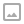## Python - Numbers

·   ·  ☕ 5 min read

Python Number Types: int, float, complex

Python includes three numeric types to represent numbers: integers, float, and complex number.

Int

In Python, integers are zero, positive or negative whole numbers without a fractional part and having unlimited precision, e.g. 0, 100, -10. The followings are valid integer literals in Python.

>>> 0
0
>>> 100
100
>>> -10
10
>>> 1234567890
1234567890
>>> y=5000000000000000000000000000000000000000000000000000000
5000000000000000000000000000000000000000000000000000000


Integers can be binary, octal, and hexadecimal values.

>>> 0b11011000 # binary
216
>>> 0o12 # octal
10
15


All integer literals or variables are objects of the int class. Use the type() method to get the class name, as shown below.

>>>type(100)
<class 'int'> # type of x is int

>>> x=1234567890
>>> type(x)
<class 'int'> # type of x is int

>>> y=5000000000000000000000000000000000000000000000000000000
>>> type(y) # type of y is int
<class 'int'>


Leading zeros in non-zero integers are not allowed e.g. 000123 is invalid number, 0000 is 0.

>>> x=01234567890
SyntaxError: invalid token


Python does not allow comma as number delimiter. Use underscore _ as a delimiter instead.

>>> x=1_234_567_890
>>> x
1234567890


Note that integers must be without a fractional part (decimal point). It it includes a fractional then it becomes a float.

>>> x=5
>>> type(x)
<class 'int'>
>>> x=5.0
>>> type(x)
<class 'float'>


The int() function converts a string or float to int.

>>> int('100')
100
>>> int('-10')
-10
>>> int('5.5')
5
>>> int('100', 2)
4


Binary

A number having 0b with eight digits in the combination of 0 and 1 represent the binary numbers in Python. For example, 0b11011000 is a binary number equivalent to integer 216.

>>> x=0b11011000
>>> x
216
>>> x=0b_1101_1000
>>> x
216
>>> type(x)
<class 'int'>


Octal

A number having 0o or 0O as prefix represents an octal number. For example, 0O12 is equivalent to integer 10.

>>> x=0o12
>>> x
10
>>> type(x)
<class 'int'>


A number with 0x or 0X as prefix represents hexadecimal number. For example, 0x12 is equivalent to integer 18.

>>> x=0x12
>>> x
18
>>> type(x)
<class 'int'>


Float

In Python, floating point numbers (float) are positive and negative real numbers with a fractional part denoted by the decimal symbol . or the scientific notation E or e, e.g. 1234.56, 3.142, -1.55, 0.23.

>>> f=1.2
>>> f
1.2
>>> type(f)
<class 'float'>


Floats can be separated by the underscore _, e.g. 123_42.222_013 is a valid float.

>>> f=123_42.222_013
>>> f
12342.222013


Floats has the maximum size depends on your system. The float beyond its maximum size referred as “inf”, “Inf”, “INFINITY”, or “infinity”. Float 2e400 will be considered as infinity for most systems.

>>> f=2e400
>>> f
inf


Scientific notation is used as a short representation to express floats having many digits. For example: 345.56789 is represented as 3.4556789e2 or 3.4556789E2

>>> f=1e3
>>> f
1000.0
>>> f=1e5
>>> f
100000.0
>>> f=3.4556789e2
>>> f
345.56789


Use the float() function to convert string, int to float.

>>> float('5.5')
5.5
>>> float('5')
5.0
>>> float('     -5')
-5.0
>>> float('1e3')
1000.0
>>> float('-Infinity')
-inf
>>> float('inf')
inf


Complex Number

A complex number is a number with real and imaginary components. For example, 5 + 6j is a complex number where 5 is the real component and 6 multiplied by j is an imaginary component.

>>> a=5+2j
>>> a
(5+2j)
>>> type(a)
<class 'complex'>


You must use j or J as imaginary component. Using other character will throw syntax error.

>>> a=5+2k
SyntaxError: invalid syntax
>>> a=5+j
SyntaxError: invalid syntax
>>> a=5j+2j
SyntaxError: invalid syntax


Arithmetic Operators

The following table list arithmetic operators on integer values:
Operator Description Example

• (Addition) Adds operands on either side of the operator. »> a=10; b=20
>>> a+b
30

• (Subtraction) Subtracts the right-hand operand from the left-hand operand. »> a=10; b=20
>>> b-a
10
>>> a-b
-10

• (Multiplication) Multiplies values on either side of the operator. »>
a=10; b=20
>>> a*b
200


/ (Division) Divides the left-hand operand by the right-hand operand.

>>> a=10; b=20
>>> b/a
2


% (Modulus) Returns the remainder of the division of the left-hand operand by right-hand operand. »> a=10; b=22

>>> b%a
2


** (Exponent) Calculates the value of the left-operand raised to the right-operand. »> a=3

>>> a**2
9
>>> a**3
27


// (Floor Division) The division of operands where the result is the quotient in which the digits after the decimal point are removed. But if one of the operands is negative, the result is floored, i.e., rounded away from zero (towards negative infinity): »> a=9; b=2

>>> a//b
4


Arithmetic Operations on Complex Numbers

Addition and subtraction of complex numbers is straightforward. Real and imaginary parts are added/subtracted to get the result.

>>> a=6+4j
>>> a+2
(8+4j)
>>> a*2
(12+8j)
>>> a/2
(3+2j)
>>> a**2
(20+48j)
>>> b=3+2j
>>> a+b
(9+6j)
>>> a-b
(3+2j)


The arithmetic operators can also be used with two complex numbers, as shown below.

>>> a=6+4j
>>> b=3+2j
>>> a+b
(9+6j)
>>> a-b
(3+2j)
>>> a*b
(10+24j)


The process of multiplying these two complex numbers is very similar to multiplying two binomials. Multiply each term in the first number by each term in the second number.

a=6+4j
b=3+2j
c=a*b
c=(6+4j)*(3+2j)
c=(18+12j+12j+8*-1)
c=10+24j


### Type Casting

A numeric object of one type can be converted in another type using the following functions:

Built-in FunctionDescription
intReturns the integer object from a float or a string containing digits.
floatReturns a floating-point number object from a number or string containing digits with decimal point or scientific notation.
complexReturns a complex number with real and imaginary components.
hexConverts a decimal integer into a hexadecimal number with 0x prefix.
octConverts a decimal integer in an octal representation with 0o prefix.
powReturns the power of the specified numbers.
absReturns the absolute value of a number without considering its sign.
roundReturns the rounded number.WRITTEN BY
Ohidur Rahman Bappy
📚Learner 🐍 Developer